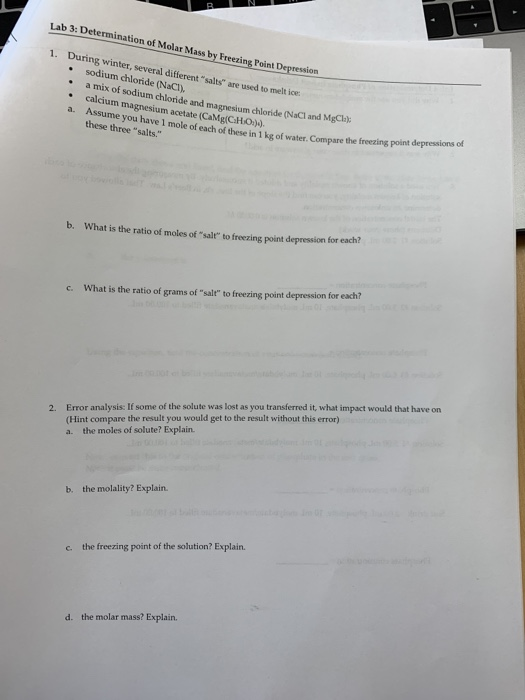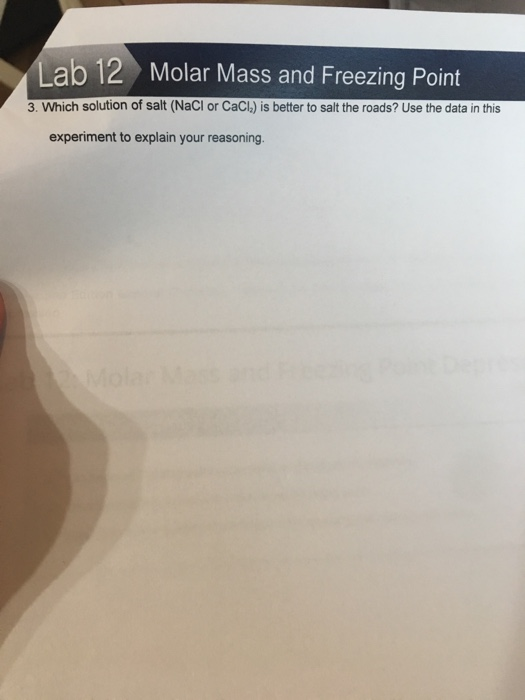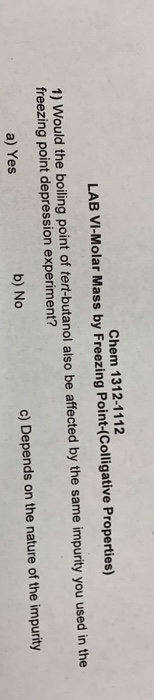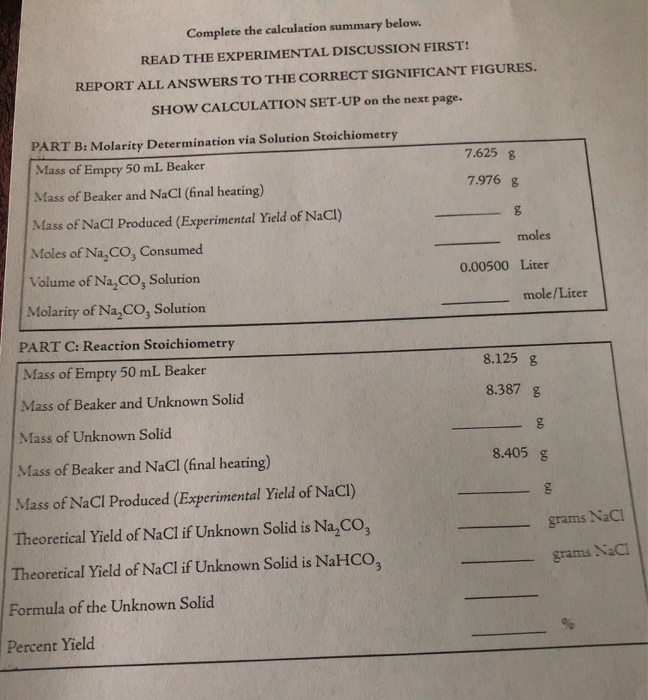# Lab 3: Determination of Molar Mass by Freezing Point Depression 1 During winter, several different "salts"...Lab 3: Determination of Molar Mass by Freezing Point Depression 1 During winter, several different "salts" are used to melt ice sodium chloride (NaCI), a mix of sodium chloride and magnesium chloride (NaCl and MgCla calcium magnesium acetate (CaMg(CHO)). Assume you have 1 mole of each of these in 1 kg of water. Compare the freezing point depressions of these three "salts." a. b. What is the ratio of moles of "salt" to freezing point depression for each? What is the ratio of grams of "salt" to freezing point depression for each? 01 C. Error analysis: If some of the solute was lost as you transferred it, what impact would that have on oe 2. (Hint compare the result you would get to the result without this error) the moles of solute? Explain. a the molality? Explain. b. the freezing point of the solution? Explain. C d. the molar mass? Explain.

a)

Freezing point depression constant for the the water is 1.86 K.Kg/mole

molality of the the solution is

For NaCl is 1 Mole/Kg of solution

For Mixture of NaCl and MgCl2 is 2 Mole/Kg of solution

For the calcium magnessium acetate is 1 Mole/Kg of solution

the molality is same in each of the three cases so the depression in the freezing point is same for all casesKf is the freezing point depression constant

m is the molality of the solution

freezing point of pure water is 273.15 Khence the depression in the freezing point is 1.86 K for the NaCl and Calcium Magnesium Acetate solution

new freezing point of the solution is 273.15-1.86=271.29 K

For MgCl2 and NaCl both are one mole

= 1.86*2 = 3.72 K

the new freezing point is 273.15 - 3.72 = 269.43 K

for the case where all three are present in the solution hence the concentration of the solution now becomes 3 moles/Kg

new freezing point depression is

=1.86*4 K

= 7.44 K

new freezing point is 273.15 - 7.44 = 265.71 K

b)

ratio of moles of salts to the boiling point depressionc)a)

As the only solute is lost hence only solute moles will decrease

b)

As the solvent is not lost only solute is lost hence molarity as well as molality will decrease

c)

As the molality is decreasing because of decrease in moles of the solute so freezing point depression will also decrease

d)

molar mass is the constant quantity so the it will not decrease because molar mass for one mole of substance is constant

##### Add Answer of: Lab 3: Determination of Molar Mass by Freezing Point Depression 1 During winter, several different "salts"...
Similar Homework Help Questions
• ### Ab 12 Molar Mass and Freezing Point 3. Which solution of salt (NaCI or CaCl) is better to salt th...ab 12 Molar Mass and Freezing Point 3. Which solution of salt (NaCI or CaCl) is better to salt the roads? Use the data in this experiment to explain your reasoning 2 Molar Mass and Freezing Point CHM1 e the Molar Mass of Each Compound Glycerol зн , s "y 03 @gvoY(sx1) (3시.):3ut & +чた Naci 2 malarmas LNac Table 1: Freezing Point Temperatures Solution Freezing Point (C) но Glycerol-3.s NaCI CaCl Post-Lab Questions ab 12 Molar Mass and Freezing...

• ### Molecular Mass by Freezing point Depression (Preliminary Lab) The following errors occurred when the above experiment was carried out

Molecular Mass by Freezing point Depression (Preliminary Lab) The following errors occurred when the above experiment was carried out. How would each affect the calculated molecular mass of the solute (too high,too low, no effect)? Explain your answer. (a) The thermometer uses actually read 1.4C too high. (b) Some of the solvent was spilled before the solute was added.

• ### Chem 1312-1112 LAB VI-Molar Mass by Freezing Point-(Colligative Properties) 1) Would the boiling point of tert-butanol...Chem 1312-1112 LAB VI-Molar Mass by Freezing Point-(Colligative Properties) 1) Would the boiling point of tert-butanol also be affected by the same impurity you used in the freezing point depression experiment? a) Yes b) No c) Depends on the nature of the impurity

• ### i uploaded everything that was given. Where would I find the experiemental values? Complete the calcula...i uploaded everything that was given. Where would I find the experiemental values? Complete the calculation summary below. READ THE EXPERIMENTAL DISCUSSION FIRST! REPORT ALL ANSWERS TO THE CORRECT SIGNIFICANT FIGURES. SHOW CALCULATION SET-UP on the next page. PART B: Molarity Determination via Solution Stoichiometry 7.625 g Mass of Empty 50 mL Beaker 7.976 g Mass of Beaker and NaCl (final heating) Mass of NaCl Produced (Experimental Yield of NaCl) moles Moles of Na,CO, Consumed 0.00500 Liter Volume of Na,CO,...

• ### I have a chemistry test that I need help with

I have a chemistry test that I need help with. My answers have stars by them Can someone please help me with the answers to this test??? Part 1 – Matter as Energy & Structure 1. When a pair of electrons is shared between two atoms, a covalent bond is said to have formed. Most often, the pair is formed a. by excess valence electrons b. as one atom contributes both electrons* c. as each atom contributes one electron d....

Need Online Homework Help?